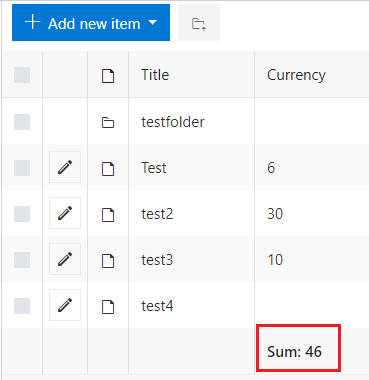# List and library control wrong total

Hi i used the below code to calculate total, but when it goes to the next page it calculate the total of that page only.

``````function calcTotal() {

var value = fd.control('SPDataTable1').widget.dataItems();
var total = 0;
if(value){
for (var i = 0; i < value.length; i++){
if(value[i].Amount)
total += parseFloat(value[i].Amount.replace(/(?!^-)[^0-9.]/g, "").replace(/(\..*)\./g, '\$1'));
}
}
fd.field('Total').value = total;
}
``````

first page total was 1000 and when i add contents to the second page the total becomes 200. The output should be 1000+200 = 1200
How do i calculate the whole total regardlsess of which page it is? and also how do i disable paging?

Hello @Rinu,

Yes, this code calculates totals only for the items that are displayed on the page.

The appearance of the related items in List or Library control depends on the List View settings. You can change the Item Limit per page in the List View settings to display all items on one page.

Also, in List View settings, you can set up displaying totals per column

So the related items will be displayed like this: